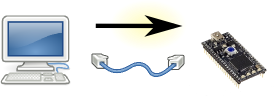# eFirmata

Connecting the real world to the digital world.

Introduction

Compatable Hardware:

Protocols:

Example Projects:

Compiler

Installing the Binary

## Protocol - PWM over UDP (port 2116)

#### PWM - Set PWMsPWMdata from the PC to the eFirmata
0001020304050607 0809101112131415 1617181920212223 2425262728293031
'e' 'F' 'i' 'r'
'm' 'a' 't' 'a'
'P' 'W' 'M' Version = 0
Reserved = 0

PWM Batch(es) ...

... (each batch is 36 octets) ...

Each batch controls up to 32 PWM values.

The number of batches is inferred from the packet length.

#### a PWM Batch

PWM batch (one batch controls 32 PWMs)
0001020304050607 0809101112131415 1617181920212223 2425262728293031
PWM PWM PWM PWM
PWM PWM PWM PWM
PWM PWM PWM PWM
PWM PWM PWM PWM
PWM PWM PWM PWM
PWM PWM PWM PWM
PWM PWM PWM PWM
PWM PWM PWM PWM

The bitmask allows us to change some PWM values, while leaving others alone. If the mask is 1, then we are setting the value, based on the data in the packet. If the mask is 0, then the eFirmata will leave that PWM alone.

The bitmask is big-endian which, sadly, means that the right-most bit (lsb) corresponds to PWM#0 (the first PWM value) and the left-most bit (msb) corresponds to to PWM#31 (the last PWM value).

Each pwm value is an unsigned, 8 bit integer. 0 is a duty cycle of 0%. 127 is a duty cycle of 49.8%. 255 is a duty cycle of 100%.

For the LPC1768 / LPC1769 microcontroller, we ignore PWM#0. We start counting at PWM#1 (going up to PWM#6). This allows us to have consistent numbering with the LPC datasheet. (And, of course, we ignore PWMs #7 through #31, because the LPC only has 6 physical outputs.)

#### Response

There is no response packet. Good luck.